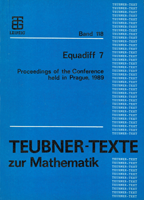Equadiff 7 Proceedings of the 7th Czechoslovak Conference on Differential Equations and Their Applications held in Prague, 1989Editor: Kurzweil, Jaroslav
Organized by: Mathematical Institute of the Czechoslovak Academy of Sciences
Venue: Prague, 1989

Publisher: BSB B.G. Teubner Verlagsgesellschaft, Leipzig, 1990
ISBN: 3322007693
Series: Teubner-Texte zur Mathematik, Bd. 118

MR1128324 | Zbl 0704.00019
MSC: 00B25, 34-06, 35-06, 65-06

 3-4 Preface.
 5-8 Contents.

Plenary lectures
 9-17 On some recent results on the numerical problems in semiconductor device simulation.  Brezzi, Franco; Marini, L. D.; Pietra, P.
 18-27 On analysis of quasihyperbolic equations of linear viscoelasticity.  Brilla, J.
 28-36 On the regularity and non-regularity of elliptic and parabolic systems.  John, O.; Stará, J.
 37-46 On some boundary value problems for systems of nonlinear ordinary differential equations.  Kiguradze, I.
 47-58 Nonlinear surface waves under external forcing.  Kirchgässner, K.
 59-70 Generalized ordinary differential equations - a survey.  Schwabik, Š.
 71-73 Oblique boundary value problems for nonlinear parabolic equations.  Uraltseva, N. N.

Section A: Ordinary differential equations
 74-76 The accuracy of numerically computed orbits of dynamical systems.  Chow, S.-N.; Palmer, K. J.
 77-79 On centers of type A and B of polynomial systems.  Conti, R.
 80-83 On the third order nonlinear ordinary differential equation.  Greguš, M.
 84-87 Nonoscillation theorems for a class of neutral functional differential equations of arbitrary order.  Jaroš, J.; Kusano, T.
 88-91 A new view of center manifolds.  Knobloch, H. W.
 92-95 Stable periodic oscillations in systems with monotonous hysteresis nonlinearity.  Krasnoselsky, M. A.; Pokrovsky, A. V.; Semenov, M. E.
 96-99 Perturbed discrete time dynamical systems.  Lasota, A.
 100-102 Stability and averaging properties of stochastic evolution equations.  Maslowski, B.
 103-105 Stability and asymptotic properties of dynamical systems in the plane.  Ráb, M.; Kalas, J.
 106-109 Multipoint boundary value problems at resonance.  Rachůnková, I.
 110-113 Theory of completely integrable equations.  Reizinsh, L.
 114-117 Relaxation oscillations in systems with different time scales.  Schneider, K. R.
 118-122 On a boundary value problem with general linear conditions.  Šeda, V.
 123-126 Linear perturbations of differential systems with constant matrices.  Šimša, J.
 127-130 Solutions to a differential inclusion of order \$n\$.  Švec, M.
 131-134 Hamiltonian systems with periodic nonlinearities.  Szulkin, A.
 135-138 Subharmonic bifurcation in equivariant systems.  Vanderbauwhede, A.
 139-142 Differential equations, higher monotonicity and principal pair of solutions.  Vosmanský, J.

Section B: Partial differential equations
 143-145 Functionals depending on curvatures and surfaces with curvature measures.  Anzellotti, G.
 146-150 Some geometric aspects of Sobolev spaces.  Bojarski, B.
 151-154 On the floating body problem.  Doppel, K.
 155-158 Solvability and bifurcations of some strongly nonlinear equations.  Drábek, P.
 159-162 Finiteness of bound states of general \$n\$-body operators.  Evans, W. D.; Lewis, R.; Saitó, Y.
 163-166 Blow up for a nonlinear degenerate parabolic equation.  Fila, M.; Filo, J.
 167-170 Homogenization and correctors for nonlinear elliptic equations.  Franců, J.
 171-174 The Dirichlet problem for sublaplacians on nilpotent Lie groups - geometric criteria for regularity.  Hansen, W.; Hueber, H.
 175-179 Solution of nonlinear degenerate elliptic-parabolic systems in Orlicz-Sobolev spaces.  Kačur, J.
 180-182 On regularity of solutions to some problems of mathematical physics.  Koshelev, A. I.; Chelkak, S. I.; Chistyakov, V. M.
 183-186 Partial differential inequalities as equations with hysteresis.  Krejčí, P.
 187-190 Pressure jumps in the dam problems.  Luckhaus, S.
 191-194 Stability of stationary solutions of parabolic variational inequalities.  Quittner, P.
 195-198 On the nodal set of eigenfunctions.  Ruf, B
 199-201 The Helmholtz decomposition and the weak Neumann problem in \$L^q\$.  Simader, C. G.
 202-203 Singular lines in liquid crystals.  Souček, J.
 204-207 On a class of scalar conservation laws with locally unbounded solutions.  Tesei, A.; Natalini, R.
 208-211 Dirichlet's problem on a snowflake.  Wallin, H.

Section C: Numerical Methods and Applications
 212-213 Eigenvalue problems with functional-differential equations.  Albrecht, J.
 214-217 On the solvability of the non-homogeneous potential problem at a corner singularity.  Arscott, F.
 218-224 Condition number estimates for elliptic difference problems with anisotropy.  Axelsson, O.
 225-227 A new iterative algorithm for solving the fictitious fluxes method problems for elliptic equations.  Bakhvalov, N. S.; Knyazev, A. V.
 228-231 On the coupling of finite elements and boundary elements for transonic potential flow computations.  Berger, H.; Warnecke, G.; Wendland, W.
 232-235 The optimal control problem in coefficients for the pseudoparabolic variational inequality.  Bock, I.; Lovíšek, J.
 236-239 Numerical calculations for some types of boundary value problems with unbounded domains.  Collatz, L.
 240-242 An electromagnetic free-boundary problem.  Descloux, J.; Maillard, P.
 243-247 Remarks to chaotic vibration in nonlinear dynamical systems.  Fazekas, F.
 248-251 Finite element solution of nonlinear elliptic equations with discontinuous coefficients and approximations in Sobolev-Slobodeckij spaces.  Feistauer, M.; Ženíšek, A.
 252-255 Quasi-Newton updates in row-methods for avoiding Jacobians.  Grossmann, Ch.
 256-259 Domain optimization in axisymmetric elliptic problems.  Hlaváček, I.
 260-264 Numerical aspects of computation of periodic and quasiperiodic solutions in systems of partial differential equations.  Holodniok, M.; Kubíček, M.
 265-270 Numerical modelling of tube fixing in the heat exchanger of a nuclear power plant.  Hřebíček, J.; Polcar, P.
 271-274 Some remarks to numerical solution of the Euler and Navier-Stokes equation.  Kozel, K.
 275-278 Local superconvergence analysis of the approximate boundary-flux calculation.  Lazarov, R. D.; Pehlivanov, A. I.
 279-280 Extrapolation of finite element solutions on non-uniform quadrilateral meshes.  Lin, Qun
 281-284 Uniqueness of the Rothe method for the Burgers equation with piecewise continuous initial data.  Martensen, E.
 285-288 A finite element method for a model of population dynamics with spatial diffusion.  Milner, F.
 289-292 The global solvability to the equations of motion of viscous gas with an artificial viscosity.  Neustupa, J.
 293-296 Transfer of boundary conditions for two-dimensional problems.  Práger, M.; Taufer, J.; Vitásek, E.
 297-300 On the mathematical and numerical modelling of electron beams.  Raviart, P.-A.
 301-304 On an internal approximation of a class of elliptic eigenvalue problems.  Vanmaele, M.; van Keer, R.
 305-308 Some features of the nodal recovery of gradients from finite element approximations which produces superconvergence.  Whiteman, J. R.; Goodsell, G.

 309-312 List of invited speakers.